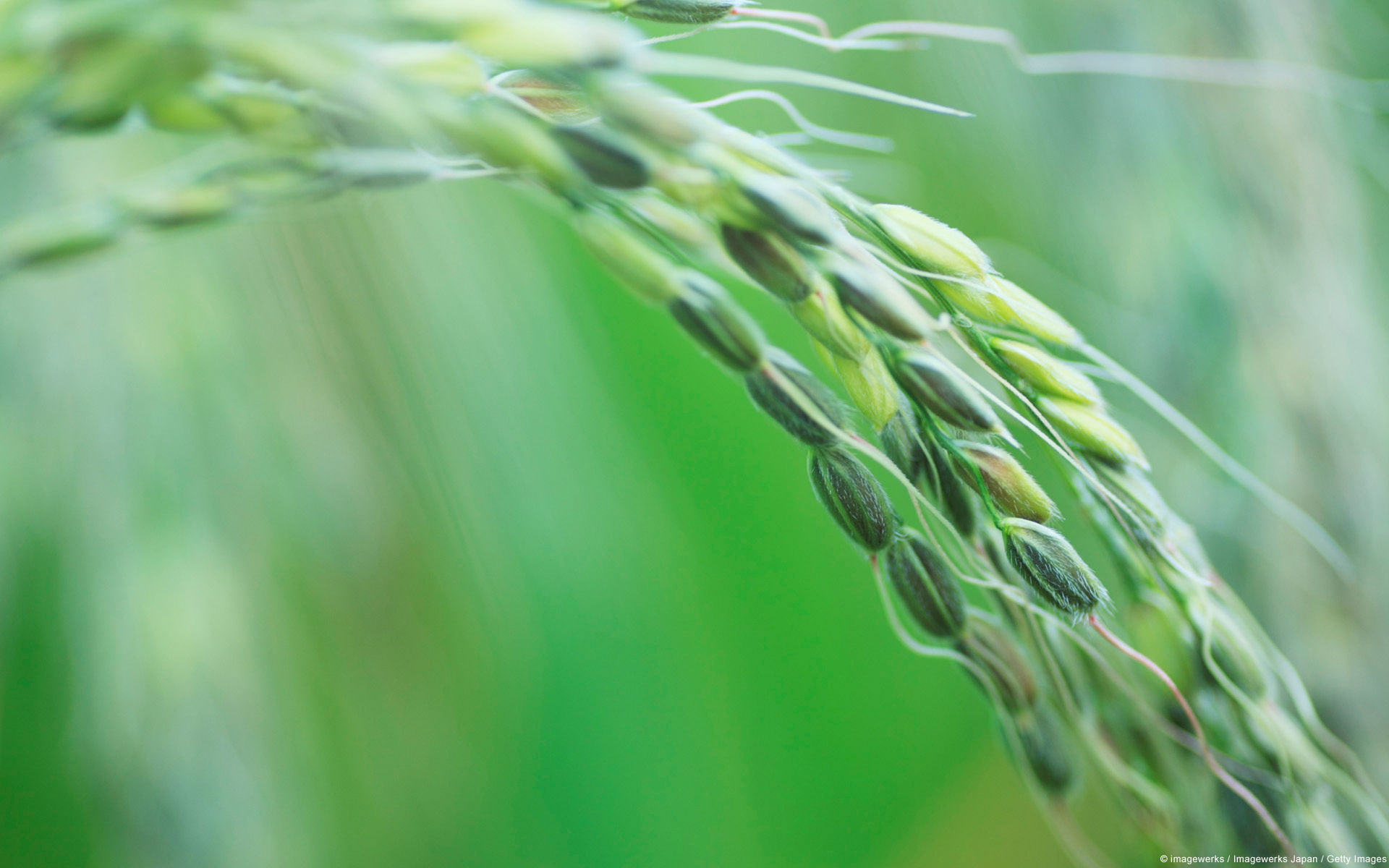top of pageWEIGHT &  MEASURES

TEMPERATURE

To convert degrees Celsius or Centigrade to degrees Fahrenheit:

Multiply by 9,divide by 5, then add 32.

To convert degrees Fahrenheit to degrees Celsius or Centigrade:

Subtract 32, Multiply by 5,then divide by 9.

-10  °C  =  14  °F                          20  °C  =   68  °F

0  °C  =  32  °F                          30  °C  =   86  °F

5  °C  =  41  °F                          40  °C  = 104  °F

10  °C  =  50  °F                        100  °C  = 212  °F

VOLUME

1 cu Centimeter   =                 1,000   cu  millimeters

1 cu Decimeter    =                 1,000   cu  centimeters

1 cu Meter           =                 1,000   cu  decimeters

CAPACITY

Metric Units

1 Centiliter       =    10            milliliters

1 Liter              =   100           centiliter                =    1000      milliliters

Imperial Units

1 US   gill          =       5            fluid ounce

1 US   pint        =       4            gill                         =     0.4732   Liter

1 US   quart     =       2            pints                      =     0.9464   Liter

1 US   gallon    =      4            quart                      =     3.7854   Liter

1 US   peck      =       2            gallon

1 US   bushel   =       4            peck

1 US   quarter  =       4            bushels

1 US   bulk barrel = 36           gallon

WEIGHT

1 Ounce             =     28.35      grams

1 Pound             =     16           ounce                  =     453.6       grams

1 Kilogram         =       2.204    pounds                =       35.26     ounce

1 Quarter           =     12.70      kilograms            =        28          pounds

1 Hundred wt     =       4           quarters

1 Long Tons       =       1.016    metric tonnes      =       20          hundred wt.

1 Short Tons      =      0.9072   metric tonnes

LENGTH

1 Inches             =      2.540     centimeters        =         25.40     millimeters

1 Foot                =    12          inches

1 Yard                =      3            feet

1 Meters            =      1.0936   yards                   =           3.2808   feet

1 Miles               =      16093    meters                =      1760           yards

1 Miles               =      1.6093   kilometers

AREA

1 Sq Inches        =         6.4516   Sq Centimeters

1 Sq Feet           =     929.03       Sq  Centimeters

1 Sq Meter         =         1.196     Sq Yards        =    10.76      Sq feet

1 Hectare           =     100            are                 =      2.471     Acres

1 Sq  Kilometer  =     100            Hectares

1 Sq Miles          =         2.590     Sq Kilometers

bottom of page